# Understanding Computer Vision : Part 1

Text book definition of pixel normally is considered the “color” or the “intensity” of light that appears in a given place in our image. If we think of an image as a grid, each square contains a single pixel.

pixels are the raw building block of an image, there is no finer granularity than the pixel. Most pixels are represented in two ways:

1. Grayscale/single channel
2. Color

Lets take an example image :Fig 1.1 This Image is 4000 pixels wide and 3000 pixels tall, total = 4000 * 3000 = 1,20,00,000 pixels

The image in Fig 1.1 has 4000 pixels wide and 3000 pixels tall, total = 4000 * 3000 = 1,20,00,000 pixels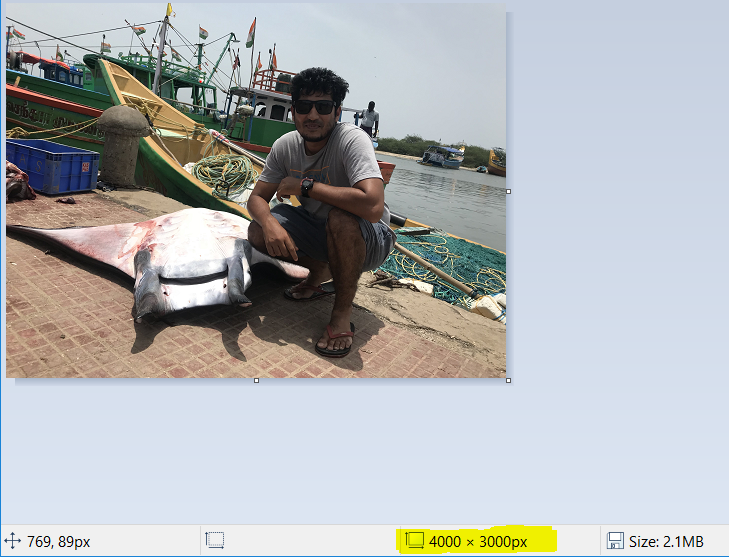Fig 1.2 Image opened with MS Paint to find the pixels of image

You can find the pixels of your image by opening the image in MS Paint and find the width * height of the images in pixels by checking the description of image as highlighted(Yellow) in the Fig 1.2 . There are also other ways of finding the pixels of image using openCV , which we will discuss in the upcoming sections of this article.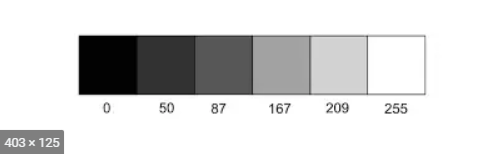Fig 1.3 Grayscale Image with values 0 to 255. 0 = Black and 255 = White

In a Grayscale or single channel image, each pixel is a scalar value between 0 to 255, where 0 = “Black” and 255= “white”. As shown in the Fig 1.3, the values closer to 0 are darker and values closer to 255 are lighter.

On the other hand, Color images are represented in RGB (Red,Green and Blue). Here the pixels values are represented by a list of three values:one value for the Red component, one for Green, and another for Blue.

R in RGB — values defined in range of 0 to 255

G in RGB — values defined in range of 0 to 255

B in RGB — values defined in range of 0 to 255

All three components combines in an additive color space to form a colored single pixel usually represented as a tuple (red, green, blue). For example consider the color“white”–we would ﬁll each of the red, green, and blue buckets up completely, like this: (255, 255, 255). Then, to create the color black, we would empty each of the buckets out (0, 0, 0), as black is the absence of color. To create a pure red color, we would ﬁll up the red bucket (and only the red bucket) completely: (255, 0, 0).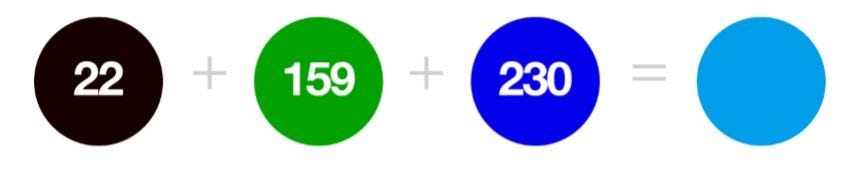Fig 1.4 R has value 22, G has value 159, B has value 230 combined in an additive manner to result in Navy Blue color component

We can conceptualize an RGB image as consisting of three independent matrices of width W and height H, one for each of the RGB components. We can combine these three matrices to obtain a multi-dimensional array with shape W×H×D where D is the depth or number of channels(for the RGB color space, D=3)

OK ! Back to basics again, Image is represented as a grid of pixels. Assume the grid as a piece of graph paper. Using this graph paper, the origin point (0,0) corresponds to the upper-left corner of the image. As we move down and to the right, both the x and y values increase. As shown in the below Fig 1.5, The letter “L” placed on a piece of graph paper. Pixels are accessed by their (x,y)coordinates, where we go x columns to the right and y rows down, keeping in mind that Python is zero-indexed.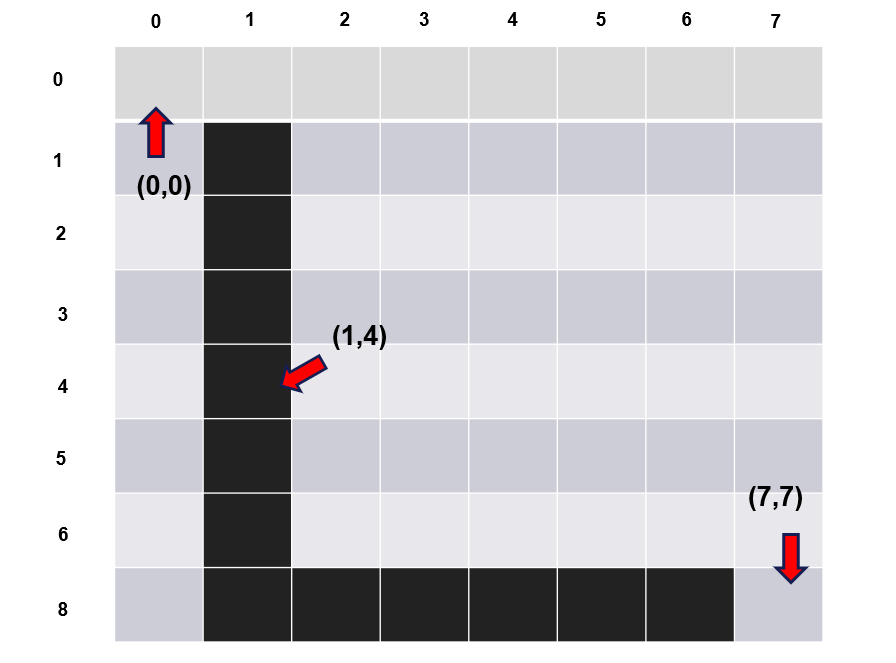FIG 1.5 LETTER “L” ON A PIECE OF OUR GRAPH PAPER. WE SEE THAT THIS IS AN 8×8 GRID WITH A TOTAL OF 64 PIXELS

## Images as NumPy array:

Image processing libraries such as OpenCV and scikit-image represent RGB images as multidimensional NumPy arrays with shape (height, width, depth). It has to be noted that the height comes first and the width due to the matrix notation. When deﬁning the dimensions of matrix, we always write it as rows x columns. The number of rows in an image is its height whereas the number of columns is the image’s width. The depth will still remain the depth. Depth indicates if the image is a color or grayscale image. If the depth is 1, then the image is a grayscale. While depth of 3 indicates it as a colored RGB image.

Let’s write a small python program to understand how to read an image using OpenCV library and extract the shape of the image.

OpenCV (Open Source Computer Vision Library) is an open source computer vision and machine learning software library. OpenCV library has more than 2500 optimized algorithms, which includes a comprehensive set of both classic and state-of-the-art computer vision and machine learning algorithms. These algorithms can be used to detect and recognize faces, identify objects, classify human actions in videos, track camera movements, track moving objects etc…

For readers who are new to setting up a python environment, follow the python setup article (click on the link) to setup python in your local machine. Follow the instructions as it is.

For this small python program, i will be using notepad++ and windows command prompt to execute the program.

After installation of python. Open command prompt and install OpenCV library using the below command.

pip install opencv-python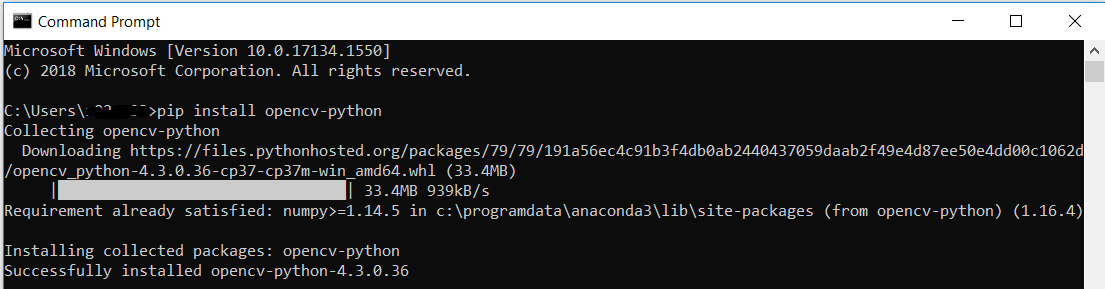Fig 1.6 Installing OpenCV library in local machine

After successfully installing the OpenCV library, Open any editor of your choice or notepad++ and type the below lines of code.

#Reading Image from disk

import cv2
print(f’(Height,Width,Depth) of the image is: {image.shape}’)
cv2.imshow(“Image”, image)
cv2.waitKey(0)

Here we load an image named example.jpg from disk and display it to our screen. My terminal output follows: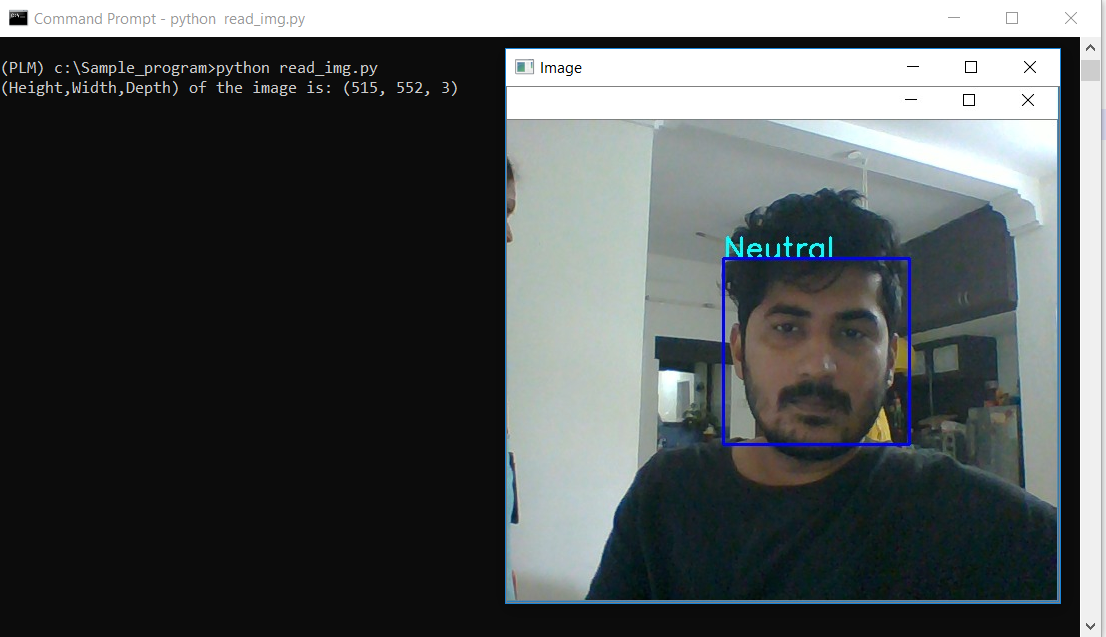Fig 1.7 Result showing the shape of Image in (Height, Width, Depth)

This image has a width of 552 pixels (the number of columns), a height of 515 pixels (the number of rows), and a depth of 3 (the number of channels).

To access an individual pixel value from our image we use simple NumPy array indexing: Copy paste the below python code in your editor and execute.

import cv2
# Receive the pixel co-ordinate value as [y,x] from the user, for which the RGB values has to be computed
[y,x] = list(int(x.strip()) for x in input().split(‘,’))
# Extract the (Blue,green,red) values of the received pixel co-ordinate
(b,g,r) = image[y,x]
print(f’ The Blue Green Red component value of the image at position [y,x]:{(y,x)} is: {(b,g,r)}’)

The above program outputs :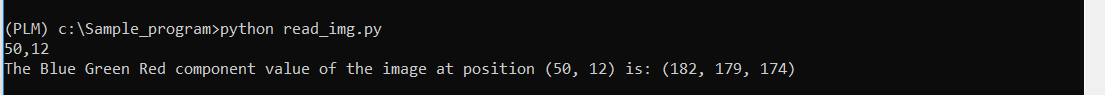Fig 1.8 The (b,g,r) value of pixel at position (50,12) is (182,179,174)

Again, notice how the y value is passed in before the x value — this syntax may feel uncomfortable at ﬁrst, but it is consistent with how we access values in a matrix: ﬁrst we specify the row number then the column number. From there, we are given a tuple representing the Blue, Green, and Red components of the image.

We can validate the above output using MS Paint as follows,

Ok ! lets find the color of my lips in the below image: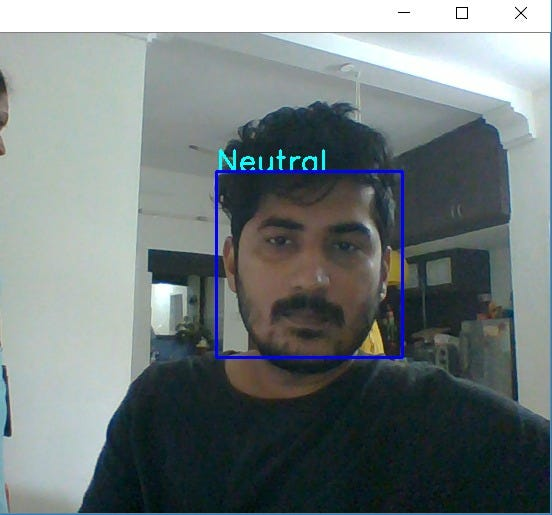Fig 1.8 Find color of lips in the image

Open the image in MSPaint and place the cursor on “lips” by checking the pixels co-ordinate (x, y) on the bottom left (highlighted in yellow) as shown below Fig 1.9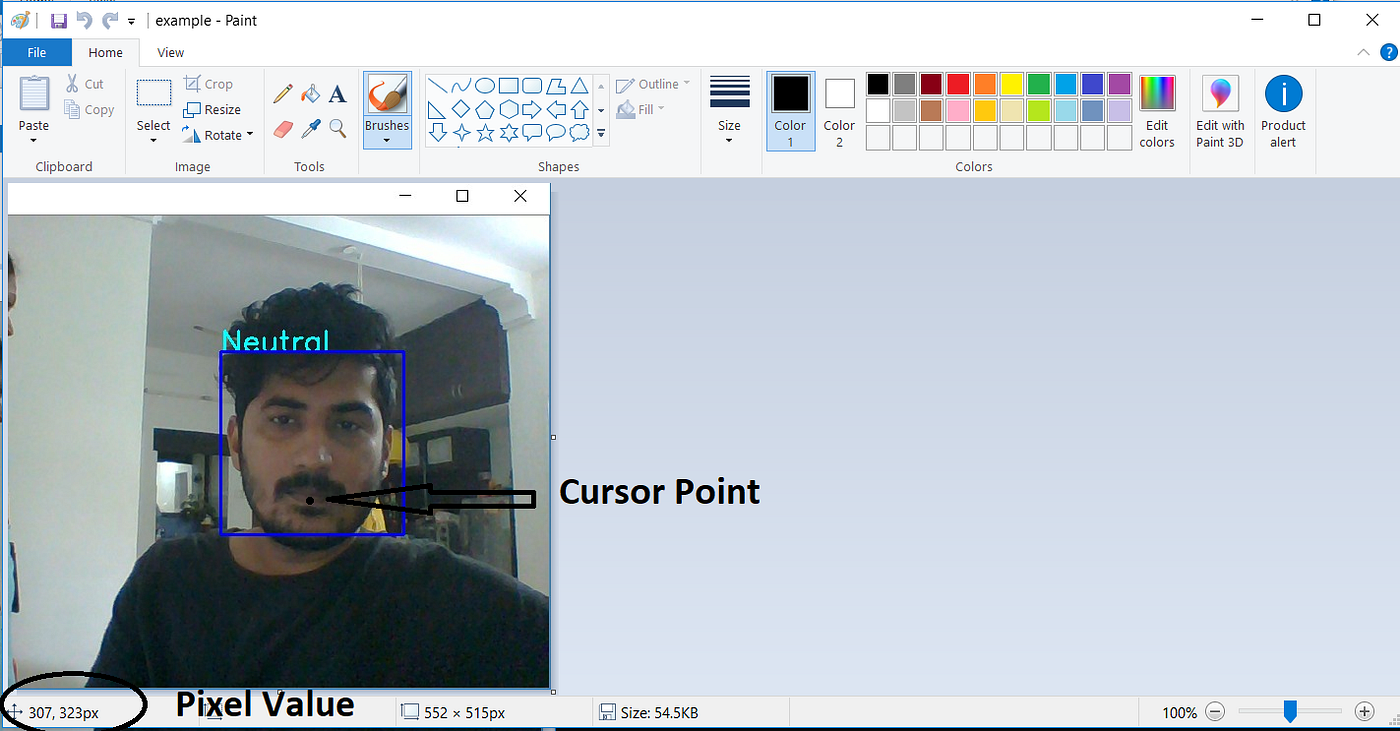Fig 1.9 Find pixel co-ordinate of my lips in the image

Input the obtained pixel co-ordinate to the python program and extract the RGB values and open Edit Colors in MS Paint to enter the obtained RGB values and validate the color of in edit colors against the image pixel co-ordinate point as shown in Figure 1.10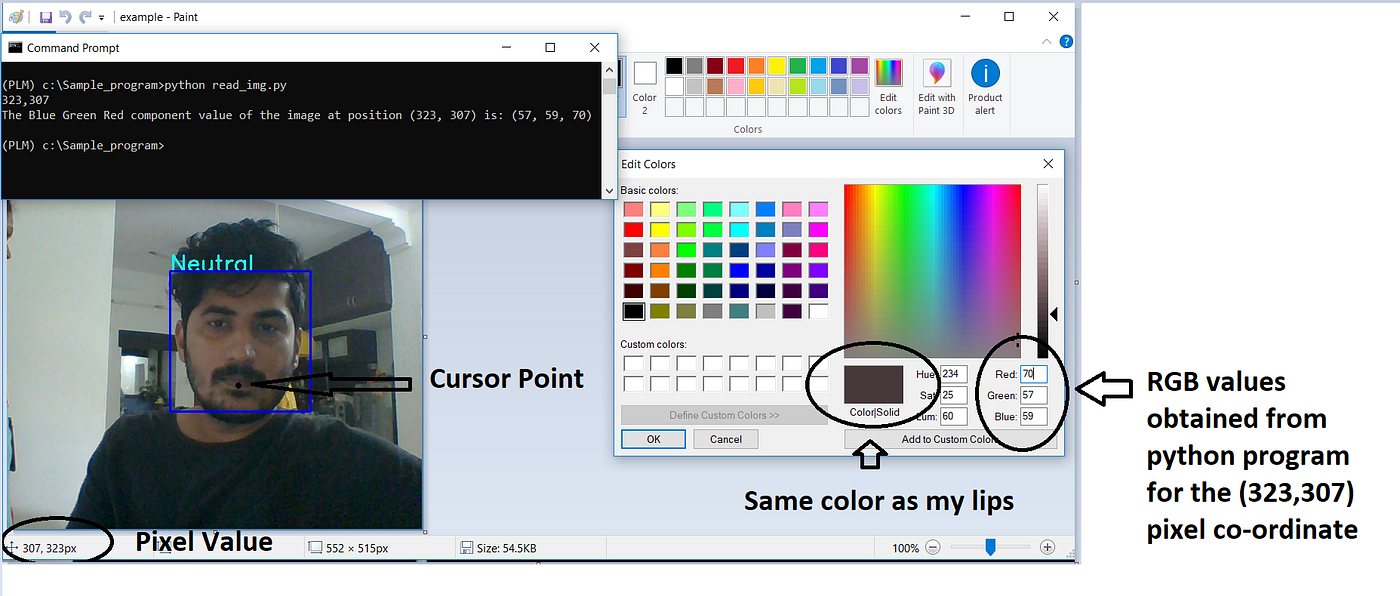Fig 1.10 Validate color of my lips by physically examining from the color obtained from Edit Colors of MS Paint

It has to be noted that, MS Paint gives the pixel co-ordinates in (x,y) opposite to the OpenCV convention and similarly RGB values are also upside down as compared to the OpenCV output format of BGR.

## Bonus Reading :

Image representation uses 4 type of data types — Unsigned character : type 1, int : type 2, float : type 4 and complex : type 8, with Unsigned Charter being the most popular as shown in the below table. The number representation on the right as shown in the Figure 1.11 are the number of bytes we use to store pixels in the memory.

Note: 1 Byte is collection of 8 Bits

So for a 28 x 28 image total pixels would be 28 x 28 = 784 pixels and the total memory for type 1 datatype would be 1B * 784 = 784 Bytes, meaning one Bytes of memory is required to store one pixel of the image.

Similarly, for a type 4 datatype (float) the same image would occupy 4B * 784 = 1568 Bytes, meaning two bytes of memory is required to store one pixel of the image.

To read the other Lessons from this course, Jump to this article to find the complete syllabus and table of content

# Don’t forget to give us your 👏 !

--

--## BoT

Bay of Tech : ”Affordable technology solutions to everyone” |BoT provides solutions in Industry 4.0 | This space is the perspective page of BoT